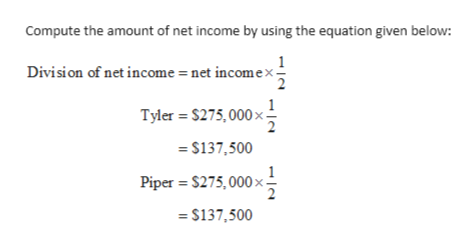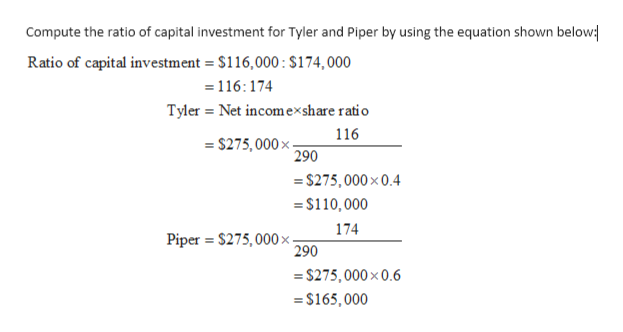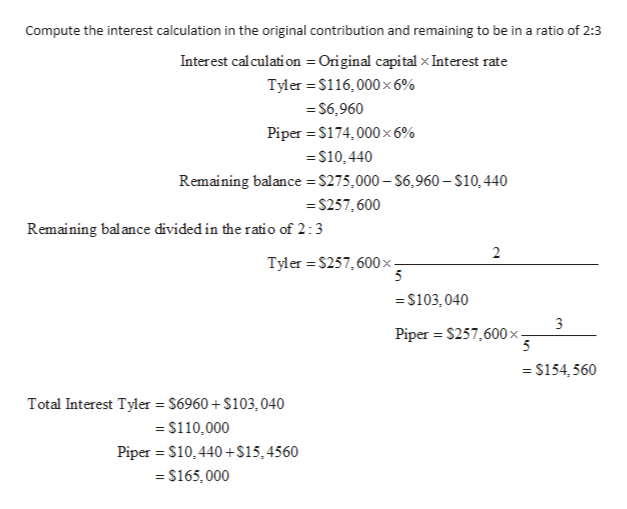# Dividing Partnership IncomeTyler Hawes and Piper Albright formed a partnership, investing \$116,000 and \$174,000, respectively.Determine their participation in the year's net income of \$275,000 under each of the following independent assumptions:No agreement concerning division of net income.Divided in the ratio of original capital investment.Interest at the rate of 6% allowed on original investments and the remainder divided in the ratio of 2:3.Salary allowances of \$38,000 and \$49,000, respectively, and the balance divided equally.Allowance of interest at the rate of 6% on original investments, salary allowances of \$38,000 and \$49,000, respectively, and the remainder divided equally.HawesAlbright(a)\$\$(b)\$\$(c)\$\$(d)\$\$(e)\$\$

Question
75 views

Dividing Partnership Income
Tyler Hawes and Piper Albright formed a partnership, investing \$116,000 and \$174,000, respectively.
Determine their participation in the year's net income of \$275,000 under each of the following independent assumptions:
No agreement concerning division of net income.
Divided in the ratio of original capital investment.
Interest at the rate of 6% allowed on original investments and the remainder divided in the ratio of 2:3.
Salary allowances of \$38,000 and \$49,000, respectively, and the balance divided equally.
Allowance of interest at the rate of 6% on original investments, salary allowances of \$38,000 and \$49,000, respectively, and the remainder divided equally.

Hawes
Albright
(a)
\$
\$
(b)
\$
\$
(c)
\$
\$
(d)
\$
\$
(e)
\$
\$

check_circle

Step 1

The division of net income has been shown in above equation. Where the net income is divided in both partners.help_outlineImage TranscriptioncloseCompute the amount of net income by using the equation given below: Division of net income = net incomex. Tyler \$275,000x =\$137,500 1 Piper \$275,000x =\$137,500 fullscreen
Step 2

The ratio of capital investment has been shown in the above equation, the net income is divided in their share ratio of capital contribution.help_outlineImage TranscriptioncloseCompute the ratio of capital investment for Tyler and Piper by using the equation shown below Ratio of capital investment \$116,000: S174, 000 =116: 174 Tyler Net incomexshare ratio 116 =S275,000x- 290 \$275,000x 0.4 =\$110,000 174 Piper \$275,000 x 290 S275,000x0.6 \$165,000 fullscreen
Step 3

In the above equation,  the interest calculation for both partners has been sho...help_outlineImage TranscriptioncloseCompute the interest calculation in the original contribution and remaining to be in a ratio of 2:3 Interest calculati on Original capital x Interest rate Tyler \$116,000x 6% = S6,960 Piper \$174,000 x 6% =\$10,440 Remaining balance \$275,000-\$6.960- \$10,440 =S257,600 Remaining balance divided in the ratio of 2:3 2 Tyler \$257,600x- 5 =S103,040 Piper \$257,600 x - =\$154,560 Total Interest Tyler \$6960+ \$103,040 =\$110,000 Piper S10,440+\$15,4560 \$165,000 fullscreen

### Want to see the full answer?

See Solution

#### Want to see this answer and more?

Solutions are written by subject experts who are available 24/7. Questions are typically answered within 1 hour.*

See Solution
*Response times may vary by subject and question.
Tagged in